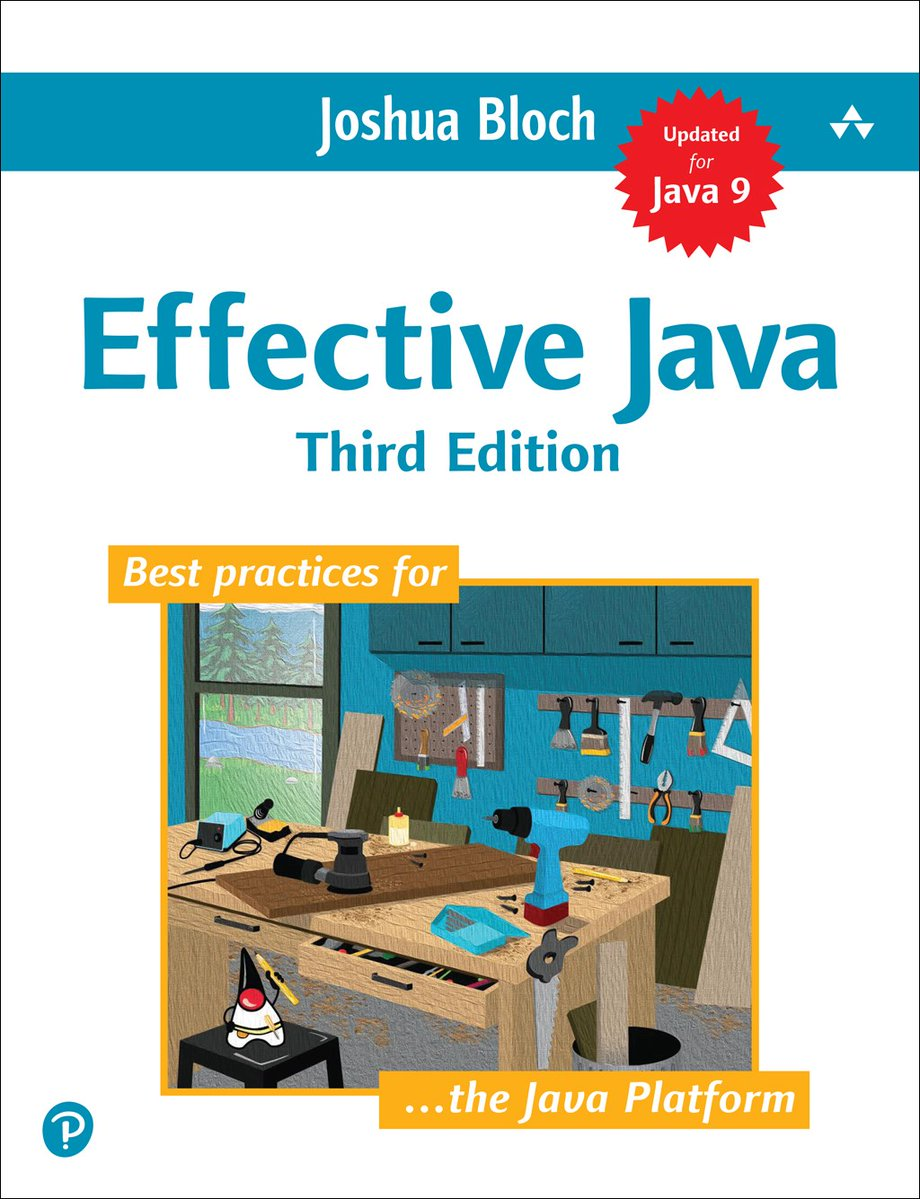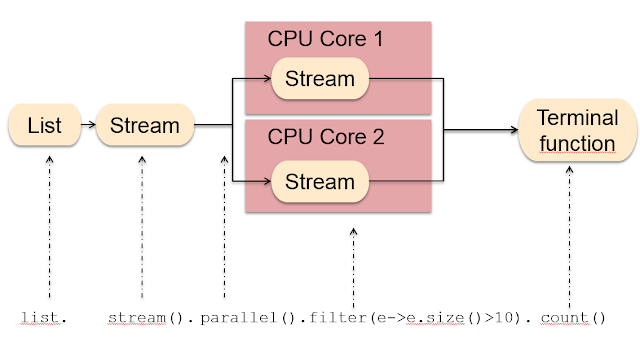# Java 8 Map, Filter, and Collect Examples

### How to use the Map and Filter functions in Java 8 to transform and filter elements of objects.

· Java Zone · Tutorial
Save
597.40K Views

Hello, guys! Many of my readers emailed me, asking to write a post about the map and filter function of Java 8, because they found it difficult to use and understand. Even though I have previously blogged about both the map() and filter(), I am writing again to expand on the concept in layman's language to provide even better understanding for everyone.

The map() function is a method in the Stream class that represents a functional programming concept. In simple words, the map() is used to transform one object into other by applying a function.

That's why the Stream.map(Function mapper) takes a function as an argument. For example, by using the map() function, you can convert a list of String into a List of Integer by applying the Integer.valueOf() method to each String on the input list.

All you need is a mapping function to convert one object to the other. Then, the map() function will do the transformation for you. It is also an intermediate Stream operation, which means you can call other Stream methods, like a filter, or collect on this to create a chain of transformations.

The filter method, as its name suggests, filters elements based upon a condition you gave it. For example, if your list contains numbers and you only want numbers, you can use the filter method to only select a number that is fully divisible by two.

The filter method essentially selects elements based on a condition you provide. That's why the filter (Predicate condition) accepts a Predicate object, which provides a function that is applied to a condition. If the condition evaluates true, the object is selected. Otherwise, it will be ignored.

Similar to map, the filter is also an intermediate operation, which means you can call other Stream methods after calling the filter.

The filter() method is also lazy, meaning it will not be evaluated until you call a reduction method, like collect, and it will stop as soon as it reaches the target.  If you are not familiar with Stream behavior, I suggest you check out The Ultimate Java 8 Tutorial, which further explains Stream fundamentals in great detail.

## 1. How to Use Map and Filter in Java 8

You need a good example to understand any new concept. Since String and Integer are the most common data type in Java, I have chosen an example that is both simple and interesting.

I have a list of String: numbers e.g. {"1", "2", "3", "4", "5", "6"}. I want to process this list and need another List of Integer with just even numbers.

In order to find the even numbers, I first need to convert a List of String to a List of Integer. For that, I can use the map() method of java.util.Stream class. But, before that, we need a Stream as a map() as defined in the java.util.stream class.

This is not difficult at all, since you can get the stream from any collection, e.g. List or Set, by calling the stream() method, which is defined in the java.util.Collection interface.

The map(Function mapper) method takes a Function, technically speaking, an object of java.util.function.Function interface. This function is then applied to each element of Stream to convert it into the type you want.

Because we need to convert a String to an Integer, we can pass either the Integer.parseInt() or Integer.valueOf() method to the map() function.

I have chosen the valueOf() method because of performance and caching. (By the way, it's not just me. Even Joshua Bloch has advised preferring static factory methods like valueOf() over constructor in Effective Java.)The map() will then return a Stream of Integer that contains both even and odd numbers. To select just even numbers, we can use the filter() method.

It takes a predicate object which is technically a function to convert an object to boolean. We pass an object and it will return true or false. The filter, then uses that information to include the object in the result stream.

To include only even numbers, we call filter( number -> number%2==0), which means each number will be divided by two, and if there is no remainder, it will be selected. This is the same logic we have used while solving coding problems to check if a given number is even or odd in Java.

We are almost done. But, so far, we only have the Stream of even integers — not the List of even Integers and that's why we need to use them.

Since we need a List, I called collect(Collectors.toList()), which will accumulate all even numbers into a List and return.

Now, you may be thinking: how does it know to return List of Integer? Well, we need to get that information by Type inference, because we have already specified that information by storing the result into a List<Integer>.

If you want to know more about type inference in a lambda expression, The Complete Java MasterClass is a good place to start.## 2. Java 8 Map + Filter + Collect Example

Here is the Java program to implement what I covered in the above section. You can run this program in IDE or from the command line and see the result.

You can also experiment with using more map() functions or more filter() calls to make the composition longer and more sophisticated. You can even play with the collect() method to collect the result in a list, set, map or any other collection.

``````package tool;

import java.util.Arrays;
import java.util.List;
import java.util.stream.Collectors;

/**
*
* A simple Java Program to demonstrate how to use map and filter method Java 8.
* In this program, we'll convert a list of String into a list of Integer and
* then filter all even numbers.
*/
public class Hello {

public static void main(String[] args) {

List<String> numbers = Arrays.asList("1", "2", "3", "4", "5", "6");
System.out.println("original list: " + numbers);

List<Integer> even = numbers.stream()
.map(s -> Integer.valueOf(s))
.filter(number -> number % 2 == 0)
.collect(Collectors.toList());

System.out.println("processed list, only even numbers: " + even);

}

}

Output
original list: [1, 2, 3, 4, 5, 6]
the processed list, only even numbers: [2, 4, 6]``````

You can see that the original list contains numbers from 1 to 6, and the filtered list only contains even numbers, i.e. 2, 4, and 6.

The most important code in this example is the following four lines of Stream processing code:This code is starting with a map, then a filter, and finally a collect. You may be wondering whether the order will matter or not. Well, it does.

Since our filter condition requires an int variable we first need to convert Stream of String to Stream of Integer. That's why we called the map() function first.

Once we have the Stream of Integer, we can apply maths to find the even numbers. We passed that condition to filter method. If we needed to filter on String, e.g. select all string which has length > 2, then we would have called filter before map.

That's all about how to use map and filter in Java 8. We have seen an interesting example of how we can use the map to transform an object to another and how to use filter to select an object based upon condition. We have also learned how to compose operations on stream to write code that is both clear and concise.

If you enjoyed this article and want to learn more about Java Collections, check out this collection of tutorials and articles on all things Java Collections.

Topics:
java 8, java streams, functional programing, tutorial, map, filter, java

Published at DZone with permission of Javin Paul, DZone MVB.

Opinions expressed by DZone contributors are their own.

Comments